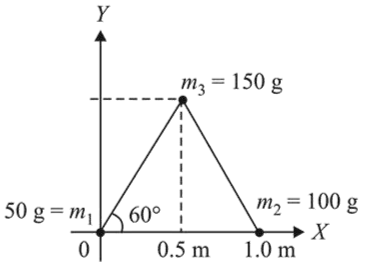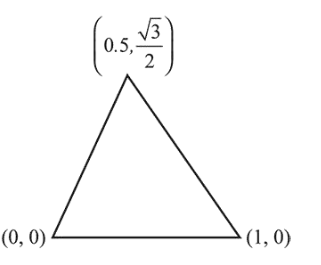# Three particles of massesQuestion:

Three particles of masses $50 \mathrm{~g}, 100 \mathrm{~g}$ and $150 \mathrm{~g}$ are placed at the vertices of an equilateral triangle of side $1 \mathrm{~m}$ (as shown in the figure). The $(x, y)$ coordinates of the centre of mass will be :1. (1) $\left(\frac{\sqrt{3}}{4} m, \frac{5}{12} m\right)$

2. (2) $\left(\frac{7}{12} m, \frac{\sqrt{3}}{8} m\right)$

3. (3) $\left(\frac{7}{12} m, \frac{\sqrt{3}}{4} m\right)$

4. (4) $\left(\frac{\sqrt{3}}{8} m, \frac{7}{12} m\right)$

Correct Option: , 3

Solution:

(3) $x_{\mathrm{cm}}=\frac{50 \times 0+100 \times 1+150 \times 0.5}{50+100+150}$

$=\frac{7}{12} \mathrm{~m}$$y_{\mathrm{cm}}=\frac{50 \times 0+100 \times 0+150 \times \frac{\sqrt{3}}{2}}{50+100+150}$

$=\frac{\sqrt{3}}{4} \mathrm{~m}$